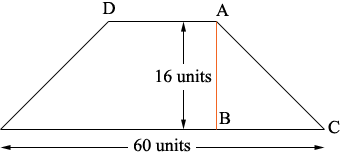SEARCH HOMEMath Central Quandaries & QueriesQuestion from Bobbi: Isosceles Trapezoid base=60"; two 45⁰ angles at base, two 135⁰ angles at the top, vertical distance between base & top=16". What is the length of the top line and the two equal side lines?Hi Bobbi,

I can get you started

I drew a diagramYou know the measure of the angle BCA is 45⁰ and the measure of the angle CAD is 135⁰. What is the measure of the angle CAB? What does that tell you about the triangle ABC? What is the length of BC?

PennyMath Central is supported by the University of Regina and The Pacific Institute for the Mathematical Sciences.